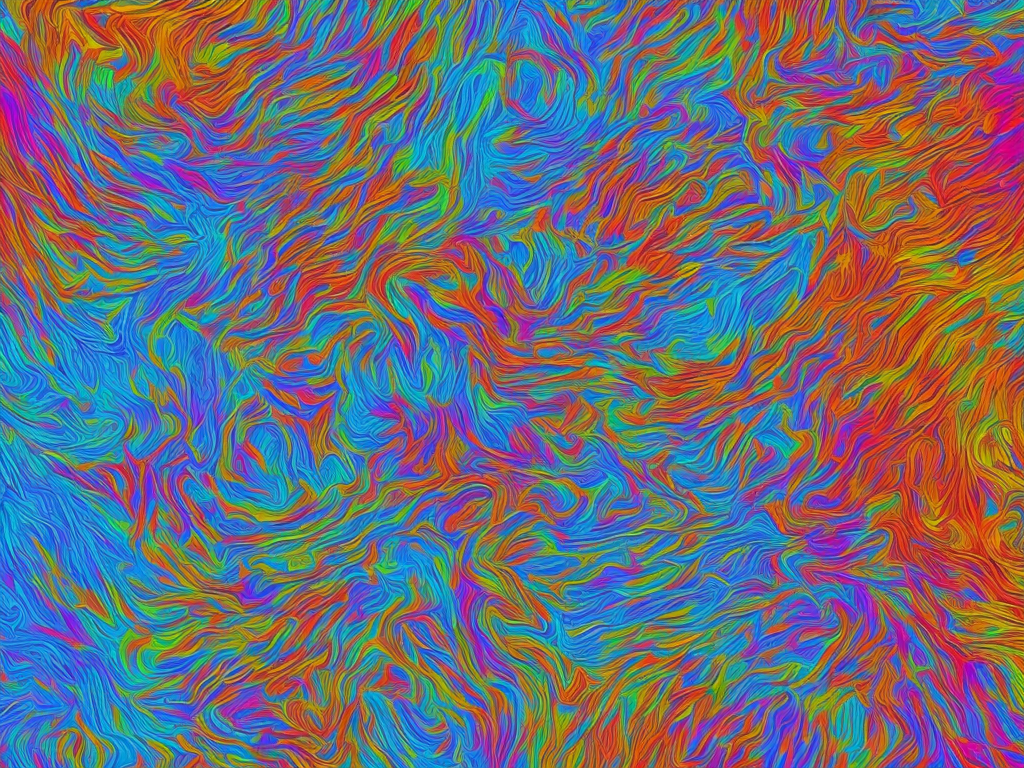# The Si Unit Of Surface Charge Density Is

tl;dr
The SI unit of surface charge density is the coulomb per square meter (C/m²).Surface charge density is an important concept in electromagnetism and plays a crucial role in a wide range of physical phenomena, including electrostatics, electrodynamics, and electrochemistry. It refers to the amount of electric charge that is distributed over a surface area, and is typically measured in units of coulombs per square meter (C/m²).

The SI unit of surface charge density is the coulomb per square meter (C/m²), which represents the amount of electric charge (in coulombs) that is distributed over a surface area (in square meters). This unit is derived from the fundamental SI unit of electric charge, which is the coulomb (C), and the fundamental SI unit of area, which is the square meter (m²).

To understand the concept of surface charge density in more detail, it is helpful to first consider the concept of electric charge. In electromagnetism, electric charge is a fundamental property of all matter that gives rise to the electromagnetic force. There are two types of electric charge: positive and negative. Like charges repel each other, while opposite charges attract each other.

In the case of surface charge density, we are interested in the distribution of electric charge over a two-dimensional surface. This could be the surface of a charged conductor or an insulator that has been charged by contact with a conductor or by exposure to an electric field.

The amount of charge that is distributed over a given surface area is defined as the surface charge density. The SI unit of surface charge density, the coulomb per square meter, is derived from the fundamental units of charge and area, as noted above. Mathematically, surface charge density can be expressed as:

σ = Q / A

where σ is the surface charge density (in C/m²), Q is the charge (in coulombs), and A is the surface area (in square meters).

For example, if a charged conductor has a total charge of 1 microcoulomb (10^-6 C) and a surface area of 0.1 square meters, the surface charge density would be:

σ = Q / A

σ = (10^-6 C) / (0.1 m²)

σ = 10^-5 C/m²

This means that there is a surface charge density of 10^-5 C/m² on the conductor's surface.

Surface charge density is an important parameter in electromagnetism because it affects the behavior of electric fields and can lead to a range of physical effects, such as electric shocks, electrostatic attraction or repulsion, and the buildup of static electricity. It is also an important concept in electrochemistry, where surface charge density can affect the rates and mechanisms of chemical reactions at the interface between two phases, such as an electrolyte solution and an electrode surface.

The SI unit of surface charge density, the coulomb per square meter, is a well-defined and internationally recognized unit that allows for clear and consistent communication of this important physical parameter. By using this unit, scientists and engineers can accurately measure and compare surface charge densities across different materials and systems, and use this information to understand and manipulate the behavior of electric fields and chemical reactions.